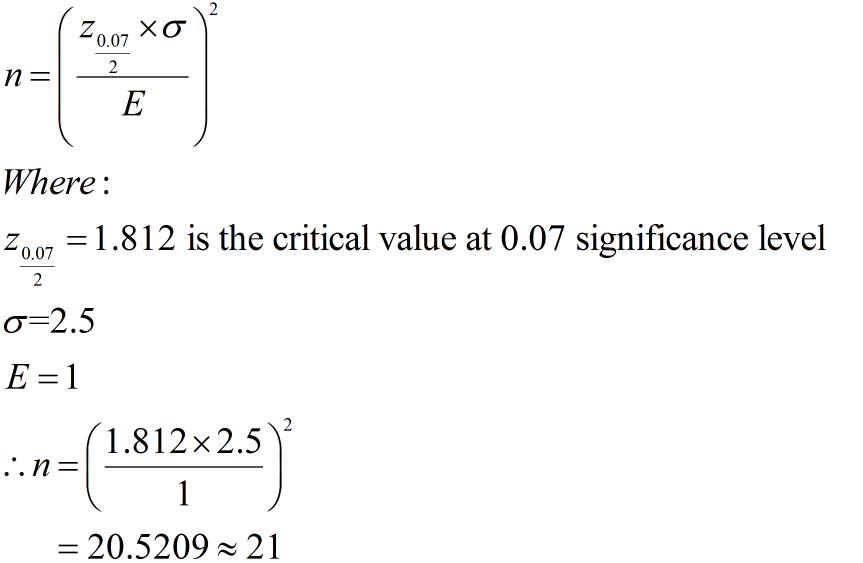# .The average height of young adult males has a normal distribution with standard deviation of 2.5 inches. You want to estimate the mean height of students at your college or university to within one inch with 93% confidence. How many male students must you measure?

Question
34 views

.

The average height of young adult males has a normal distribution with standard deviation of 2.5 inches. You want to estimate the mean height of students at your college or university to within one inch with 93% confidence. How many male students must you measure?

check_circle

Step 1

Solution: It is given that the average height of young adult males has a normal distribution with a standard deviation of 2.5 inches.

It is required to find the number of students so that the estimate of the mean height of the students at the college or university to be with one inch with a 93% confidence level.

Step 2

The formula for finding the sample size is:...

### Want to see the full answer?

See Solution

#### Want to see this answer and more?

Solutions are written by subject experts who are available 24/7. Questions are typically answered within 1 hour.*

See Solution
*Response times may vary by subject and question.
Tagged in